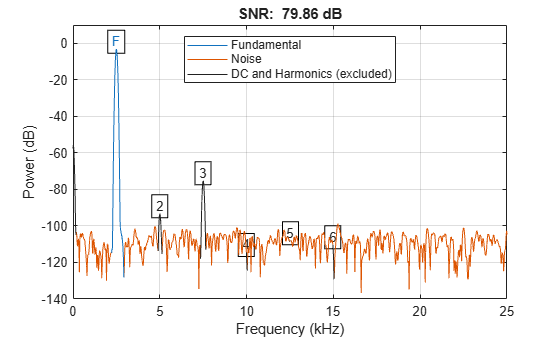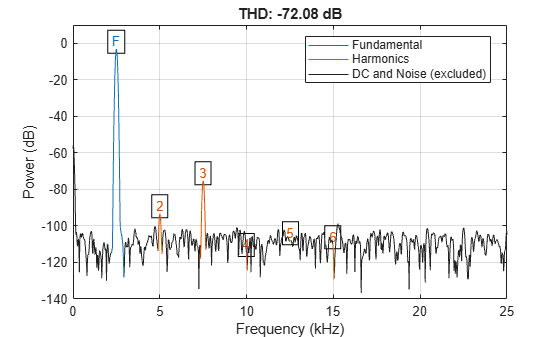# Distortion Measurements

Generate 2048 samples of a sinusoid of frequency 2.5 kHz sampled at 50 kHz. Add white Gaussian noise such that the signal-to-noise ratio (SNR) is 80 dB.

```Fs = 5e4; f0 = 2.5e3; N = 2048; t = (0:N-1)/Fs; SNR = 80; x = cos(2*pi*f0*t); x = x+randn(size(x))*std(x)/db2mag(SNR);```

Pass the result through a weakly nonlinear amplifier represented by a polynomial. The amplifier introduces spurious tones at the frequencies of the harmonics.

```amp = [1e-5 5e-6 -1e-3 6e-5 1 25e-3]; x = polyval(amp,x);```

Plot the signal spectrum and annotate the SNR, verifying that it has the expected value. The `snr` function computes the power ratio of the fundamental to the noise floor and ignores the DC component and the harmonics.

`snr(x,Fs);`Plot the signal spectrum and annotate the total harmonic distortion (THD). The `thd` function computes the power ratio of the harmonics to the fundamental and ignores the DC component and the noise floor.

`thd(x,Fs);`Plot the signal spectrum and annotate the signal to noise and distortion ratio (SINAD). The `sinad` function computes the power ratio of the fundamental to the harmonics and the noise floor. It ignores only the DC component.

`sinad(x,Fs);`Verify that the SNR, THD, and SINAD obey the equation

`$1{0}^{-SNR/10}+1{0}^{THD/10}=1{0}^{-SINAD/10}.$`

`lhs = 10^(-snr(x,Fs)/10)+10^(thd(x,Fs)/10)`
```lhs = 7.2203e-08 ```
`rhs = 10^(-sinad(x,Fs)/10)`
```rhs = 7.1997e-08 ```

Plot the signal spectrum and annotate the spurious-free dynamic range (SFDR). The SFDR is the power ratio of the fundamental to the strongest spurious component ("spur"). In this case, the spur corresponds to the third harmonic.

`sfdr(x,Fs);`